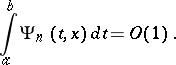# Singular integral

An integralwith a singularity at the point, defined for a functionintegrable on, whose kernel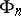satisfies the following conditions: For any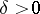and an arbitrary interval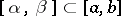,(1)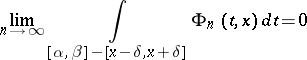(2)

and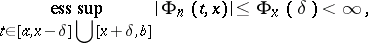(3)

where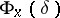depends only onandand not on. If (1), (2) and (3) are fulfilled uniformly on an-set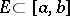, then the integral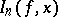is said to be uniformly singular on. Most attention has been paid to properties of so-called positive kernels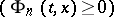; Dirichlet kernels (cf. Dirichlet kernel)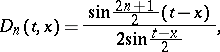Fejér kernels (cf. Fejér integral)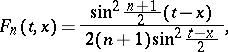Poisson–Abel kernels (cf. Poisson integral)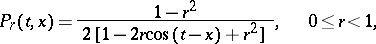and kernels induced by various methods of summation of orthogonal expansions in orthonormal polynomials.

The concept of a "singular integral" was introduced by H. Lebesgue , who pointed out its importance in the investigation of questions of convergence. Thus, the investigation of the convergence of singular integrals bears on questions of convergence and summability of trigonometric Fourier series; Fourier series in orthogonal polynomials, and also expansions in general orthogonal systems.

Lebesgue established a criterion for the convergence of singular integrals in the case of continuous functionsof bounded variation. D.K. Faddeev  established necessary and sufficient conditions for the convergence of singular integrals at a Lebesgue point in the case of summable functions. Since the conditions of Lebesgue and Faddeev are difficult to verify for concrete singular integrals, a whole series of papers was devoted to the search for effective sufficient conditions for the convergence of singular integrals, both at isolated points and uniformly. For a singular integral to converge at a continuity point, it is sufficient that the operatoris bounded in norm, i.e. the sequence of integrals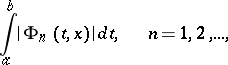must be bounded, and for convergence at a Lebesgue point it is necessary that there exists a so-called "hump-backed majoranthump-backed majorant" for the kernel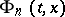, that is, an integrable function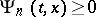which monotonically increases on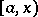and monotonically decreases on, such that for almost-all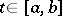,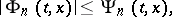where